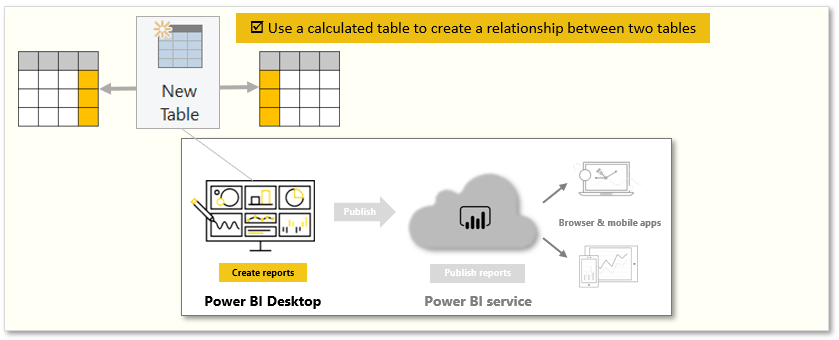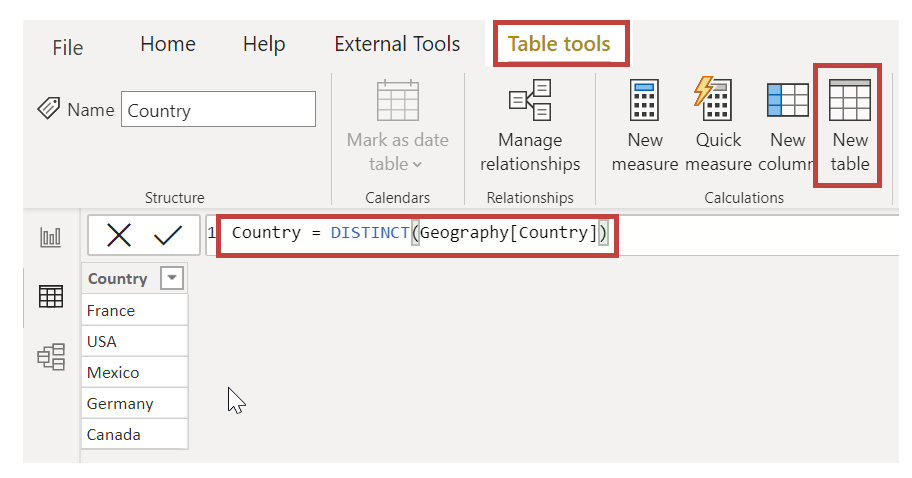# Create calculated tables

Completed

Calculated tables is a function within DAX. Most of the time, you can import data into your model from an external data source. However, calculated tables provide intermediate calculations and data that you want stored as part of the model rather than as part of a query. You can use calculated tables, for example, to cross join two tables.

Tasks in this unit include:Note